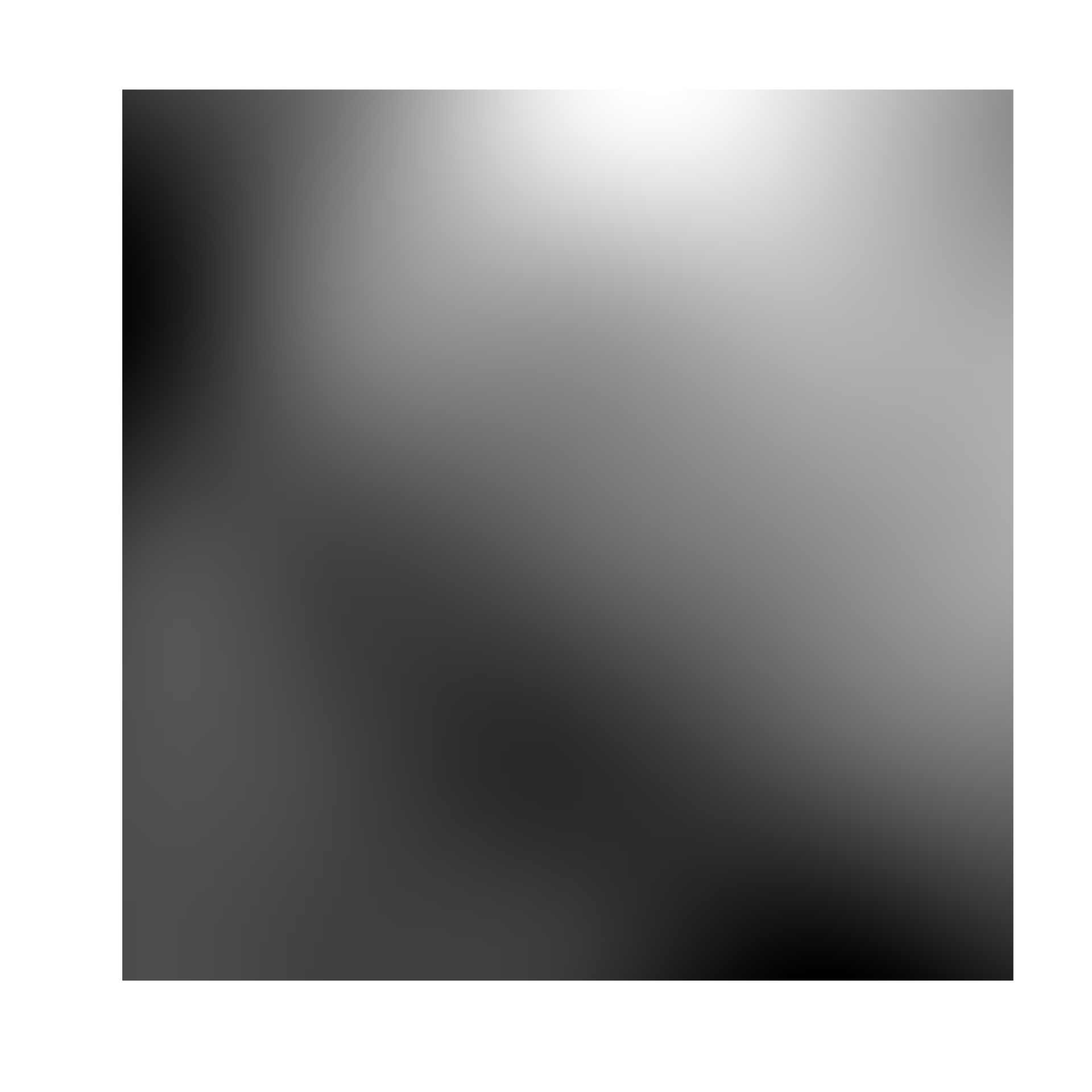Cubic noise is a pretty simple alternative to perlin and simplex noise. In essence it takes a low resolution white noise and scales it up using cubic interpolation. This approach means that while cubic noise is smooth, it is much more random than perlin and simplex noise.

noise_cubic(
dim,
frequency = 0.01,
fractal = "fbm",
octaves = 3,
lacunarity = 2,
gain = 0.5,
pertubation = "none",
pertubation_amplitude = 1
)

gen_cubic(x, y = NULL, z = NULL, frequency = 1, seed = NULL, ...)

## Arguments

dim The dimensions (height, width, (and depth)) of the noise to be generated. The length determines the dimensionality of the noise. Determines the granularity of the features in the noise. The fractal type to use. Either 'none', 'fbm' (default), 'billow', or 'rigid-multi'. It is suggested that you experiment with the different types to get a feel for how they behaves. The number of noise layers used to create the fractal noise. Ignored if fractal = 'none'. Defaults to 3. The frequency multiplier between successive noise layers when building fractal noise. Ignored if fractal = 'none'. Defaults to 2. The relative strength between successive noise layers when building fractal noise. Ignored if fractal = 'none'. Defaults to 0.5. The pertubation to use. Either 'none' (default), 'normal', or 'fractal'. Defines the displacement (warping) of the noise, with 'normal' giving a smooth warping and 'fractal' giving a more eratic warping. The maximal pertubation distance from the origin. Ignored if pertubation = 'none'. Defaults to 1. Coordinates to get noise value from The seed to use for the noise. If NULL a random seed will be used ignored

## Value

For noise_cubic() a matrix if length(dim) == 2 or an array if length(dim) == 3. For gen_cubic() a numeric vector matching the length of the input.

## Examples

# Basic use
noise <- noise_cubic(c(100, 100))

plot(as.raster(normalise(noise)))# Using the generator
grid <- long_grid(seq(1, 10, length.out = 1000), seq(1, 10, length.out = 1000))
grid$noise <- gen_cubic(grid$x, grid\$y)
plot(grid, noise)